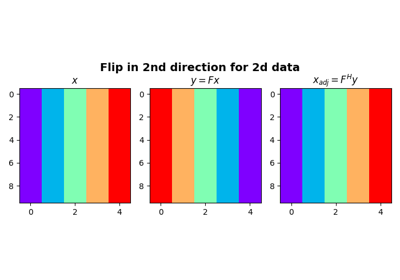# pylops.Flip¶

class pylops.Flip(N, dims=None, dir=0, dtype='float64')[source]

Flip along an axis.

Flip a multi-dimensional array along a specified direction dir.

Parameters: N : int Number of samples in model. dims : list, optional Number of samples for each dimension (None if only one dimension is available) dir : int, optional Direction along which flipping is applied. dtype : str, optional Type of elements in input array.

Notes

The Flip operator flips the input model (and data) along any chosen direction. For simplicity, given a one dimensional array, in forward mode this is equivalent to:

$y[i] = x[N-i] \quad \forall i=0,1,2,...,N-1$

where $$N$$ is the lenght of the input model. As this operator is self-adjoint, $$x$$ and $$y$$ in the equation above are simply swapped in adjoint mode.

Attributes: shape : tuple Operator shape explicit : bool Operator contains a matrix that can be solved explicitly (True) or not (False)

Methods

 __init__(self, N[, dims, dir, dtype]) Initialize this LinearOperator. adjoint(self) Hermitian adjoint. cond(self, \*\*kwargs_eig) Condition number of linear operator. conj(self) Complex conjugate operator div(self, y[, niter]) Solve the linear problem $$\mathbf{y}=\mathbf{A}\mathbf{x}$$. dot(self, x) Matrix-matrix or matrix-vector multiplication. eigs(self[, neigs, symmetric, niter]) Most significant eigenvalues of linear operator. matmat(self, X) Matrix-matrix multiplication. matvec(self, x) Matrix-vector multiplication. rmatvec(self, x) Adjoint matrix-vector multiplication. transpose(self) Transpose this linear operator.

## Examples using pylops.Flip¶Flip along an axis